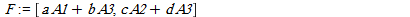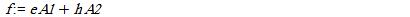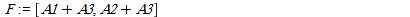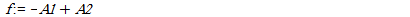# Question: Checking linearly dependent of a polynomial on a list of polynomials w.r.t. additional restrictions on parameters

## Question: Checking linearly dependent of a polynomial on a list of polynomials w.r.t. additional restrictions on parameters

I want to test linearly dependence of a polynomial f on a list of polynomials F by additional condition on parametric coefficients of linear parametric polynomial (linear for variables not parameters). Please note that:

1. The polynomialand the members of are always homogenous in the variables.
2. The coefficients of f, the coefficients of the members of F are all always polynomials in the parameters or contant and the members of N and W are all always polynomials in the parameters.

For example letand(a,b,c,d,e,h are parameters and A1,A2,A3 are variables).

If I use PolyLinearCombo(F,f,{A1,A2,A3}) (see http://www.mapleprimes.com/questions/204469-How-Can-I-Find-The-Coefficients-Of-Linear#comment217621)then its output is false,[].

Now we let to condition sets for parameters as the following:

N:=[ebc+ahd]

W:=[a,c]

The elements of N must be zero means that ebc+ahd=0

and the elements of W are non-zero that is a<>0 and c <>0.

Let a=b=c=d=h=1 and e=-1. This specialization satisfy in the above condition sets N and W. By this specialization we have:andNow if I use PolyLinearCombo(F,f,{A1,A2,A3}) then its output is true,[-1,1].

By this additional two condition sets I have to check that whether f is linearly independent of F or not. How can I do this without specialization? In fact I want an algorithm that its input is (null condition N, not-null condition W, list of polynomials F, a polynomial f, the set of variables) and its output is true and coefficients if f is linearly dependent of F w.r.t. null and not-null conditions N and W, else its output is false.

If the name of new procedure is ExtPolyLinearCombo andN:=[ebc+ahd]

W:=[a,c]

I want the output of

ExtPolyLinearCombo(N,W,F,f,{A1,A2,A3}) be true,[coefficients]

Thank you very much in advance.﻿